Home

 << Previous    1...   18  19    21  22  ...24    Next >>

20. The 24-cell embodies the holistic parameter 672

We found in #4 that, when they are Type A triangles, the faces of the 24-cell have 672 corners, sides & triangles other than their 24 vertices & 96 edges: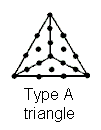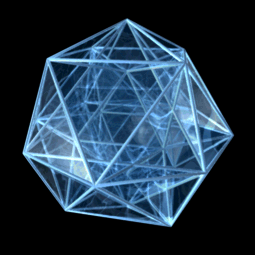The 24-cell has:

24 vertices
96 edges
96 triangular faces
24 octahedra

Numbers of geometrical elements other than
vertices & edges surrounding the centre of the 24-cell

 Corners Sides Triangles Total Faces 96 3×96=288 3×96=288 672 Interior 96 24 + 3×96 = 312 3×96=288 696 Total 192 600 576 1368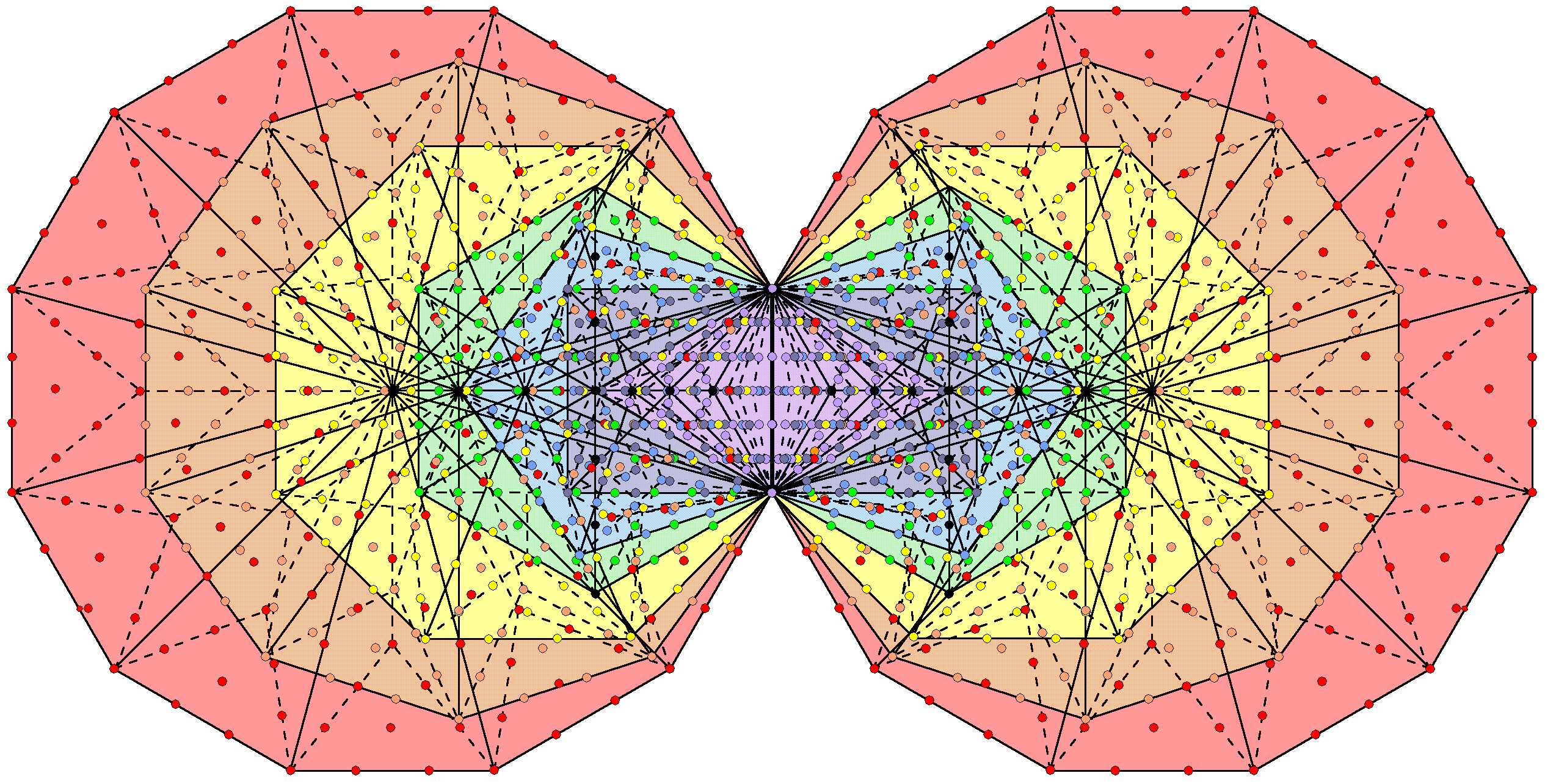Rotating 24-cell    1368 yods are intrinsic to the (7+7) Type B polygons enfolded in each overlapping Tree of Life;
12 black yods in each set of 7 polygons→12 sides of 48 internal Type A triangles in each half of the 24-cell;
672 coloured yods in each set of 7 enfolded polygons→672 geometrical elements in each half of the 24-cell.

Given how this number is embodied in the various sacred geometries discussed in previous pages, the fact that 672 extra points, lines & triangles are needed to construct the faces of the 24-cell from Type A triangles is strong evidence for its holistic nature. This is confirmed by the fact that, including the internal corners and sides of its internal triangles, 1368 geometrical elements in addition to its vertices & edges surround the centre of the 24-cell, where

 1368 = 3 + 5 + 7 + 9 +... + 73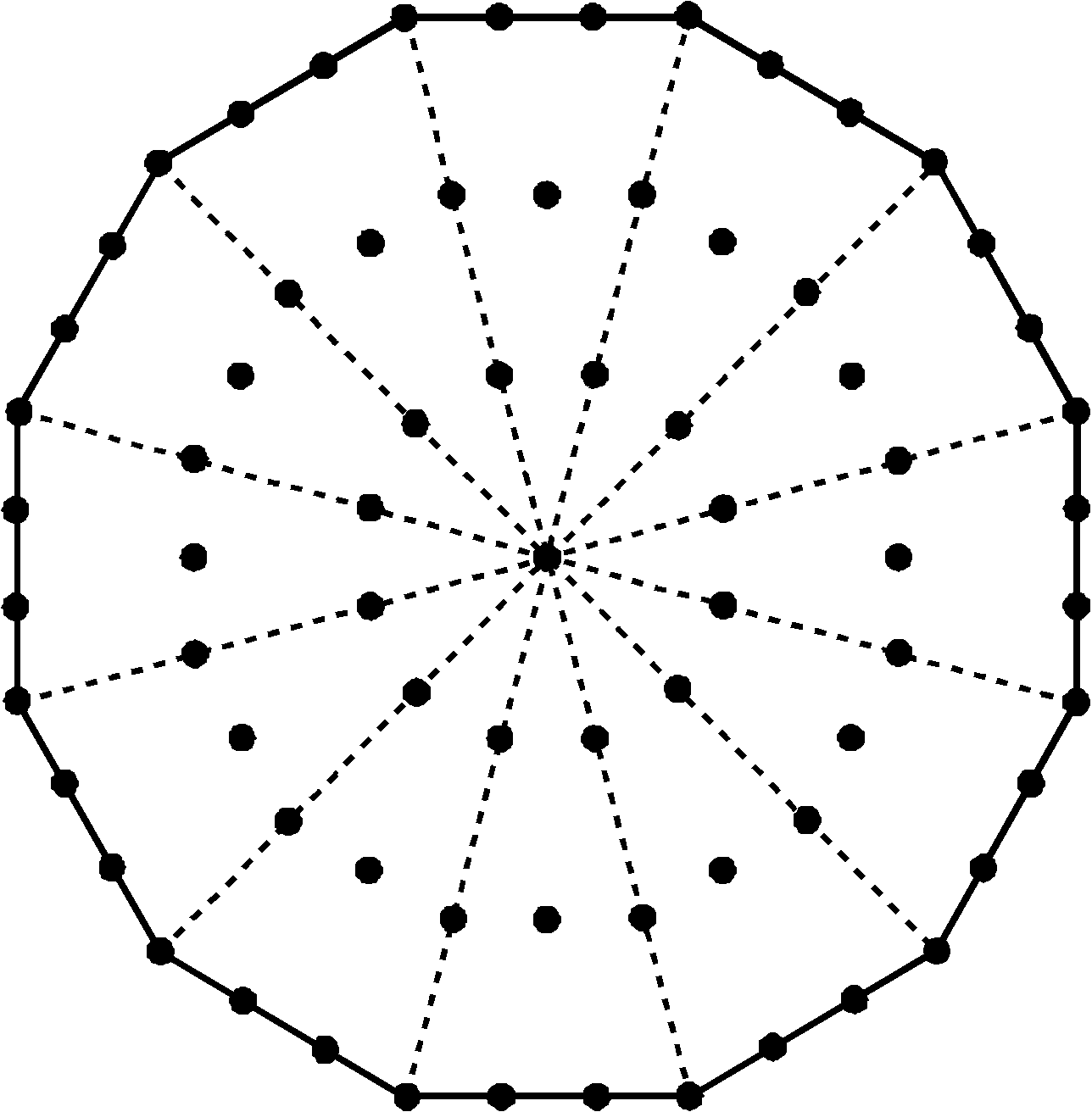The number (1368) of points, lines & triangles other than vertices & edges needed to construct the 24-cell is the sum of the first 36 odd integers after 1 up to 73. The Type A dodecagon has 73 yods. Star of David representation of the number 73 of Chokmah.

The number 73 of Chokmah ("Wisdom"), the second Sephirah of the Tree of Life, determines the geometrical composition of the 24-cell as the last of the 36 odd integers after 1 that add up to the number of geometrical elements in addition to its vertices & edges that are needed to construct the interior and exterior of the 24-cell. ELOHA, the Godname of Geburah with number value 36, prescribes in this arithmetic way how many extra geometrical elements compose the 24-cell constructed from Type A triangles. The number 73 is embodied in the Type A dodecagon as its 73 yods. It is also the number of yods needed to construct a Star of David/Sign of Vishnu from 12 tetractyses. Including the centre of the 24-cell, the number of yods on the 24 internal sides of the 96 interior Type A triangles = 1 + 24×3 = 73. As confirmation of the holistic character of the 24-cell, these extra 1368 geometrical elements are symbolised in the inner Tree of Life by the 1368 yods that are intrinsic (i.e., belonging solely) to the (7+7) Type B polygons enfolded in successive Trees of Life (see picture above). The 24 black yods that are either centres of the 14 polygons or yods lining the vertical axes of the two hexagons that are shared with the outer Tree of Life correspond to the 24 internal sides of the 96 Type A triangles inside the 24-cell formed by its 96 edges. It means that the (70+70) Type B polygons enfolded in 10 Trees of Life have 13680 yods (see Fig. 3 in #2). This is the number of yods that line the 6720 edges of the 421 polytope with 240 vertices when its 60480 faces become tetractyses: 240 + 2×6720 = 13680. It is also the number of corners, sides & triangles other than their 1200 vertices & edges that surround the common centre of the ten 24-cells in the compound of two concentric, different sized 600-cells that is the 4-dimensional projection of the 421 polytope. The 10 Trees are the Tree of Life counterpart of the ten 24-cells, the compound of the five 24-cells that form one 600-cell corresponding to the five Trees mapping the uppermost five Sephiroth of the Tree of Life and the compound of the five other 24-cells forming the second 600-cell corresponding to the five Trees that map the lowest five Sephiroth. The question that arises from this correspondence is whether these Trees individually map a single half-revolution of all 10 whorls of the UPA or whether they each map the 10 half-revolutions of a single whorl. Previously, we concluded that the 24-cells correspond to the half-revolutions of all 10 whorls because we presented evidence that the two 600-cells generate the outer and inner halves of the UPA. In either case, however, the 24-cell is corresponding to a set of 10 things — either all 10 whorls or 10 half-revolutions of a single whorl. This means that we should expect the 24-cell to display a 10-fold pattern in either its geometrical or yod composition (or in both). In the former case, it is trivial, for the 24-cell is composed of 24 vertices, (4×24=96) lines, (4×24=96) triangles & 24 octahedra, i.e., 10 sets of 24 geometrical elements.

The 24-cell has 576 triangles with 600 sides other than edges. The number of sides & triangles in the 24-cell other than edges = 600 + 576 = 1176 = 1 + 2 + 3 +...+ 48, where 48 is the number of Kokab, the Mundane Chakra of the Sephirah Hod. As

576 = 242 = 1 + 3 + 5 +...+ 47,

600 = 2 + 4 + 6 +...+ 48.

In other words, the number of sides in the 24-cell other than edges when its faces and interior triangles are Type A triangles is the sum of the first 24 even integers and the number of its triangles is the sum of the first 24 odd integers. This property illustrates the beautiful harmony between number and sacred geometry. As 1176 = 7×168 and as a circular turn in a minor whorl of the UPA comprises seven circular 2nd-order spirillae spaced evenly along its circumference (see here), this is the number of 2nd-order spirillae in every half-revolution of a minor whorl. This is not true for major whorls because every nth-order spirilla in them is composed of 7.04 (n+1)th-order spirillae, not exactly 7 (n+1)th-order spirillae. The number of sides & triangles other than edges in the ten 24-cells of the two 600-cells = 1176×10 = 7×1680, which is both the number of 2nd-order spirillae in a minor whorl and the number of 1st-order spirillae in the seven minor whorls.

Including its vertices & edges, the total number of geometrical elements surrounding the centre of the 24-cell constructed from Type A triangles = 24 + 96 + 1368 = 1488 = 31×48. Assigning the number 31 of the Godname EL ("God") to the 48 corners of the seven separate, regular polygons of the inner Tree of Life generates the number of points, lines & triangles making up the 24-cell. As the compound of five 24-cells, there are (5×1488=7440=155×48=31×240) geometrical elements surrounding the common centre of the 600-cell. In other words, assigning the number 31 of EL, the Godname of Chesed, to the 240 yods in the 1-tree that are not "Sephirothic levels" generates the number of geometrical elements in the five 24-cells whose compound is the 600-cell: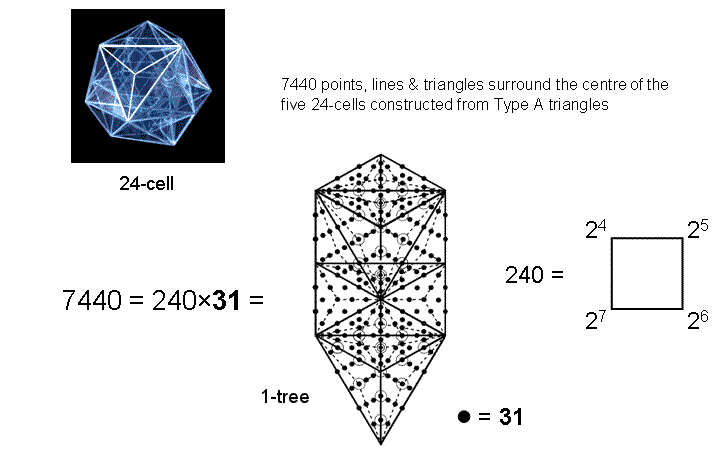1-tree representation of the 7440 geometrical elements in five 24-cells whose compound is the 600-cell.

This is how EL prescribes the number of geometrical elements making up these five 24-cells.

As 2×7440 = 14880 = 62×240, assigning the number 62 of Tzadkiel, the Archangel of Chesed, to these 240 yods generates the number of geometrical elements in the compound of two 600-cells whose 240 vertices are the 4-dimensional projection of the 240 vertices of the 421 polytope. Both the Godname and Archangel of Chesed determine the geometry of the two 600-cells! As 62×240 = 620×24 = 620×1×2×3×4, the number of geometrical elements in the ten 24-cells is 24 times the number 620 of Kether, the number of hexagonal yods in the 10-sided decagon: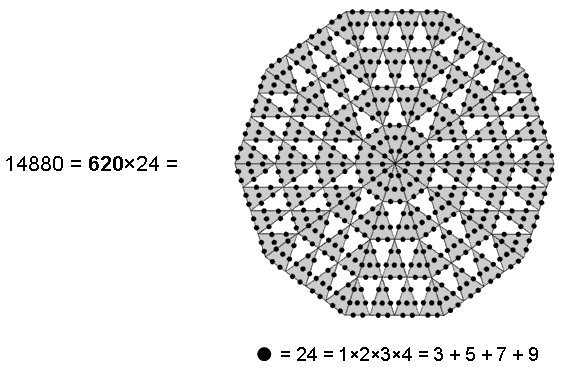Decagonal representation of the 14880 geometrical elements in the ten 24-cells in the compound of two 600-cells.

Weighted with 24 (the sum of the first 4 odd integers), the 620 hexagonal yods in the decagon with 2nd-order tetractyses as sectors generate the number of geometrical elements in the ten 24-cells of the compound of two 600-cells. Assigning the number of Kether to the 24 vertices of the 24-cell also generates this number. As 14880 = 30×496, the 30 yods lining the sides of a pentagram and weighted with the number 496 of Malkuth generate the total number of geometrical elements in the ten 24-cells: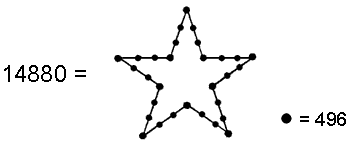496 =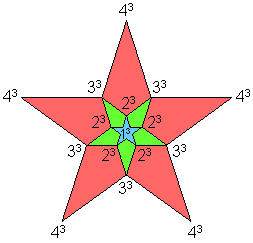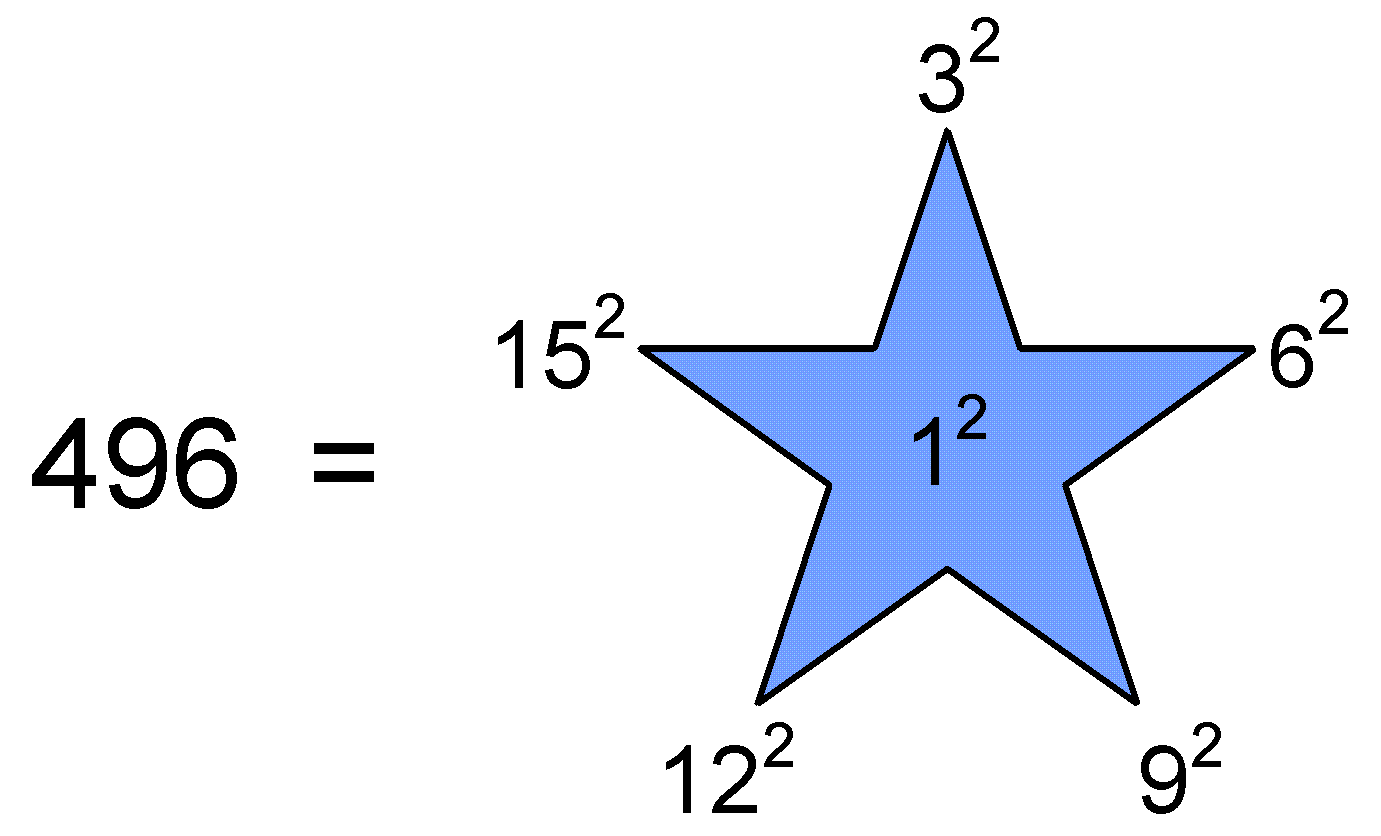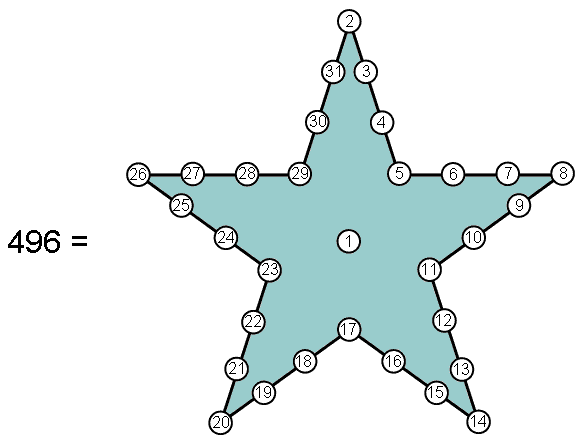The 30 yods lining a pentagram and weighted with the number 496 of Malkuth generate the total number (14880) of geometrical elements that surround the centre of the ten 24-cells in the compound of two 600-cells. 496 is the sum of 16 cubes of 1, 2, 3 & 4. 496 is the sum of the squares of 1, 3, 6, 9, 12 & 15. 496 is the sum of the first 31 integers arranged at the centre and on the boundary of a pentagram.

This demonstrates the fundamental connection between the pentagram and the geometrical composition of the ten 24-cells within the two 600-cells whose compound is the 4-dimensional projection of the 240 vertices of the 421 polytope defining the root vectors of E8.

As 14880 = 30×496 = 60×248, assigning the number 248 of Raziel, the Archangel of Chokmah, to the 60 hexagonal yods in either the Tree of Life or the dodecagon generates the total number of geometrical elements surrounding the centre of the ten 24-cells: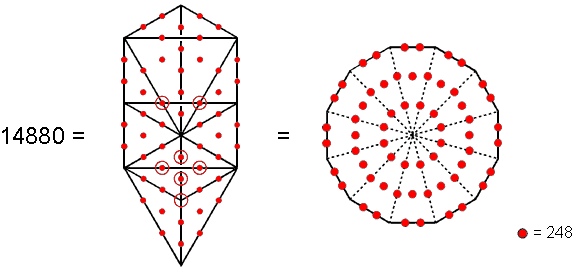Weighted with the number 248 of Raziel, the Archangel of Chokmah, the 60 hexagonal yods in the Tree of Life and in the dodecagon generate the number (14880) of geometrical elements in the ten 24-cells in the compound of two 600-cells.

The number of geometrical elements other than vertices in the faces of the 24-cell = 96 + 672 = 768 (see table above). As

384 = 22 + 32 + 42 + 52 + 62 + 72 + 82 + 92 + 102 = 48 + 336,

768 = 2×384 = 2×(22+32+42+52+62+72+82+92+10 2) = 96 + 672.

Each half of the 24-cell with 48 edges and 336 other geometrical elements conforms to the division: 384 = 48 + 336 that is characteristic of holistic systems (see The holistic pattern). The number of geometrical elements other than vertices in the faces of the five 24-cells (the compound of which is the 600-cell) = 5×768 = 3840 = 10×(22+32+42+52+62+72+82+92+10 2). This can be represented by a 10-fold array of the squares of the integers 2-10, each set of nine squares representing the number of geometrical elements other than vertices in the 48 faces of half a 24-cell: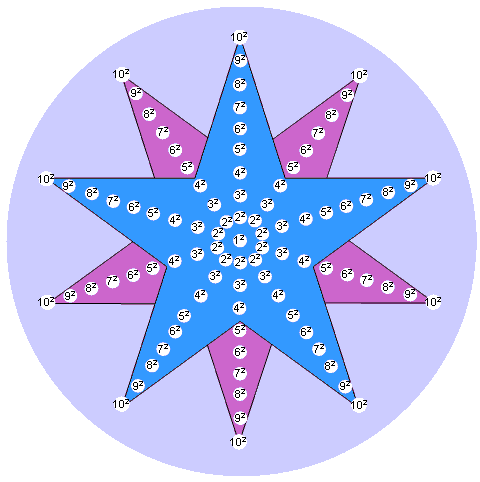The sum of the 10-fold array of the squares of the integers 2-10 is the number (3840) of geometrical elements other than vertices in the faces of the five 24-cells, the compound of which is the 600-cell. The blue pentagram denotes the upper halves of the five 24-cells and the red pentagram denotes their lower halves.

This demonstrates how the Decad determines the geometrical composition of the 600-cell as the compound of five 24-cells.

Given that the 4-dimensional projection of the 421 polytope is the compound of two 600-cells, each of which is the compound of five 24-cells, it is amazing — no, wonderful — that the number of edges (6720) of the 421 polytope is the same as the number of corners, sides & triangles in addition to their 240 vertices and 960 edges that are required to construct the 960 faces of the ten 24-cells making up the two 600-cells. As we have shown in previous pages that the number 672 is a global parameter of sacred geometries, the conclusion is inescapable that the 24-cell and the 421 polytope are holistic systems described by the universal pattern embodied in these sacred geometries, thereby indicating that the E8×E8 heterotic superstring is its microscopic manifestation, the UPA being its subquark state.

Conclusion
What has emerged from this analysis of the 24-cell is its holistic character. The fact that it is composed of 1368 geometrical elements other than its vertices & edges when its faces are Type A triangles and the fact that this is the number of yods intrinsic to the inner form of successive, overlapping Trees of Life are astounding pieces of evidence of this character that cannot be disputed. Whether the 24-cell is the geometrical basis of each whorl of the UPA or (more plausibly) each of the ten half-revolutions of all 10 whorls, the fact remains that the faces of the 24-cell contain 672 geometrical elements other than vertices & edges and that this number is a parameter of sacred geometries, being found in the first four Platonic solids, the 2-dimensional Sri Yantra and the disdyakis triacontahedron. This, alone, bestows archetypal significance to the 24-cell. That the 421 polytope has precisely ten times as many edges and that the 4-dimensional projection of its 240 vertices determining the 240 root vectors of E8 coincide with two 600-cells, each a compound of five 24-cells, is what rigorously establishes the clear link between sacred geometries and E8×E8 heterotic superstrings. That the UPA paranormally described over a century ago by Besant & Leadbeater has 6720 plane wave components in every revolution of its ten whorls is what rigorously establishes its clear link to E8×E8 heterotic superstrings. For sceptics to dismiss this connection as the result of chance is to replace the extraordinary power of micro-psi with a hypothetical coincidence so improbable as to amount to a miracle. It is not a more sensible or more rational, alternative explanation. Instead, let us see it for what it is, namely, an unrealistic, untenable way that debunkers often resort to in order to protect at all costs their ideological disbelief in anything paranormal.

See #4 for further discussion of the 24-cell.

 << Previous    1...   18  19    21  22  ...24    Next >>

Home